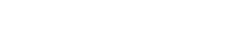# Logistic Distribution

Non Normal Distributions > Logistic Distribution

## What is a Logistic Distribution?PDF of the logistic distribution. Image: Krishnavedala| Wikimedia CommonsThe logistic distribution is used for modeling growth, and also for logistic regression. It is a symmetrical distribution, unimodal (it has one peak) and is similar in shape to the normal distribution. In fact, the logistic and normal distributions are so close in shape (although the logistic tends to have slightly fatter tails) that for most applications it’s impossible to tell one from the other.

## Why is it Used?

The logistic distribution is mainly used because the curve has a relatively simple cumulative distribution formula to work with. The formula approximates the normal distribution extremely well.

Finding cumulative probabilities for the normal distribution usually involves looking up values in the z-table, rounding up or down to the nearest z-score. Exact values are usually found with statistical software, because the cumulative distribution function is so difficult to work with, involving integration:Although there are many other functions that can approximate the normal, they also tend to have very complicated mathematical formulas. The logistic distribution, in comparison, has a much simpler CDF formula:Two parameters define the shape of the distribution:

## Other Useful Statistics

Mean (μ) = m
Variance (σ)2 = ⅓ π2 b2
Skewness (γ)1 = 0
Kurtosis (γ)2 = 6/5

Fun fact: The United States Chess Federation and the World Chess Federation (FIDE) use the logistic distribution to calculate the relative skill level of chess players. Formerly, they both used the normal distribution.

Check out our YouTube Channel for hundreds of elementary statistics and probability videos!

CITE THIS AS:
Stephanie Glen. "Logistic Distribution" From StatisticsHowTo.com: Elementary Statistics for the rest of us! https://www.statisticshowto.com/logistic-distribution/
---------------------------------------------------------------------------Need help with a homework or test question? With Chegg Study, you can get step-by-step solutions to your questions from an expert in the field. Your first 30 minutes with a Chegg tutor is free!